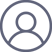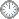李毅伟2019-4-21 11:36

注：改正了两处错误，见高亮。

This is an in-mail from TYUST.

新入の者--> What is going on ? (redirected)new

(接前：18 17 14) 温习: 命题5.7的证明.

---- 目前看清楚，配对是全篇的轴心.

---- 而配对主要是靠边界起作用.

.

---- 1. “回拉” (将配对投射到像空间).

---- 2.  造相 (涉及构造边界的技术方法).

---- 3.  准则 (配对要符合适当的奇异类型).

---- 配对即石头，边界即形状尺寸，奇异类型即颜色质地.

.

---- 核心是构造配对 (V, Γv) klt.

---- V 源于 ψ(V, X, B) ~ T.

---- 关键在于 Γv 的构造: Γv = γ·O.

γ  = (1 + t, 1 - eps/4, 1 - a).

O = (B~, E(ψ), T).

---- 原作以定义的方式给出了Γv.

---- Γv 结构较复杂，给人以突兀感.

---- 而凡是突兀出现的，必有自然的起点.

---- Γv 该是从某个地方 “倒推” 出来的.

---- 特别地，1 - eps/4 是怎么来的?

.

---- Step5 的两个公式值得注意:

a).  Kv + Γv ≡ G/X.

b).  Kv + Γv ≡ tB~ + F/X.

---- Step 4 先后给出表达式:

F:=Σ(ai - eps/4)Ei;

G:=Σ(a'i - eps/4)Ei + (a' - a)T.

---- 通常写在后面的式子是先推导出来的.

|--- 不妨猜测  Γv 来源于 a) 和 b).

---- 特别是 a)，它有“完整感”.

---- 将 a) 看做“方程”: Kv + Γv ≡ G/X.

---- 让 Γv 和 G 待定.

|--- 另一个线索是Step4末尾:

(X, B + tB) eps/2-lc, dim(ψ(Ei))>0 ==>

Ei = q[G], q > 0.

---- 结果即所需. 也许是由所需倒推出条件. (?

|--- 由Step4第一个 Kv + Γv 的表达式:

ψ*(Kx + B) = Kv + B~ + Σ(1 - ai)Ei + (1 - a)T.

---- 假定：这是个已知的公式.(?)

---- 从结构上看很像 !

---- Kx 对应 Kv; B 对应 B~ + s·Eψ.

---- Eψ = (..., Ei, ..., T).

(s·Eψ 是全部配系数超常除子之和).

---- 按这个思路， Γv 的出发点是 ψ* 项.

---- 只是由于某种原因，又添加了 tB~ + F.

|--- 用Step4第二个 Kv + Γv 式子验证假定.

---- 其中出现的 ψ*(Kx + (1 + t)B) 暂不展开.

---- 它提示另一个配对: (X, B + tB) eps/2-lc.

---- 这个 B + tB 在Step4 出现了 6次！

---- 其双有理形式 (1 + t)B~ 也出现了 2 次.

---- 推测: B + tB 是元模式.

---- 现在按假定展开 ψ*(Kx + (1 + t)B)

= Kv + (1 + t)B~ + s'·

---- 此处 s'= (..., 1 - a'i,...,1 - a').

(带撇是由于配对的 B 换成了 B + tB).

---- 对比原作，果然不错 (验证了假定).

---- 按这个思路，Γv 的出发点是 ψ* 项，但换了个配对.

----由于某种原因，又添加了 G.

.

|--- 注意到 Γv 的系数层面只有 a.

---- 没有 ai, a' 和 a'i.

(观察 Γv 表达式，对照ψ*，似乎接近了...)

---- 换句话说，要剔除带下标和撇的系数...

---- 先来看 ψ*(Kx + B) 的表达式:

B~ + Σ(1 - ai)Ei + (1 - a)T.

B~ + ΣEi + (1 - a)T - aiΣaiEi.

---- 再来看 ψ*(Kx + (1 + t)B) 的表达式:

(1 + t)B~ + Σ(1 - a'i)Ei + (1 - a')T

(1 + t)B~ + ΣEi - a'iΣa'iEi + (1 - a')T

---- 两个表达式的粉色部分取并集:

(1 + t)B~ + ΣEi + (1 - a)T

---- 此式可看作 Γv 的 “毛坯”.

---- 可以断定，Fv 的构造来源于ψ*的两个表达.

.

符号大全上下标.|| 常用：↑↓ π ΓΔΛΘΩμφΣ∈  ∪ ∩ ⊆ ⊇ ⊂ ⊃ ≤ ≥ ⌊ ⌋ ⌈ ⌉ ≠ ≡ ⁻⁰ &sup1; &sup2; &sup3; ᵈ ₀ ₁ ₂ ₃ ᵢ .

#### Glossary(AG)

*

Abstract 8/4

Introduction

....

....

11/9

...

Proposition 5.5 11/5